:   Netscrap(TM)   :   Work   :   Netscrap #896   :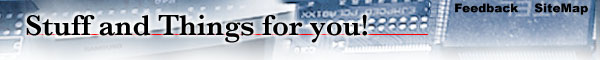What Makes 100%?

```----- Begin NetScrap(TM) -----

What Makes 100%?

What does it mean to give MORE than 100%?

Ever wonder about those people who say they are giving
more than 100%? We have all been to those meetings where
someone wants you to give over 100%.

How about achieving 103%? What makes up 100% in life?

Here's a little mathematical formula that might help you

If:
A B C D E F G H I J K L M N O P Q R S T U V W X Y Z .

is represented as:
1 2 3 4 5 6 7 8 9 10 11 12 13 14 15 16 17 18 19 20 21 22 23 24 25 26.

Then:
H-A-R-D-W-O-R-K

8+1+18+4+23+15+18+11 = 98%

and
K-N-O-W-L-E-D-G-E

11+14+15+23+12+5+4+7+5 = 96%

But,
A-T-T-I-T-U-D-E

1+20+20+9+20+21+4+5 = 100%

And,
B-U-L-L-S-H-I-T

2+21+12+12+19+8+9+20 = 103%

AND, look how far ass kissing will take you:
A-S-S-K-I-S-S-I-N-G

1+19+19+11+9+19+19+9+14+7 = 118%

So, one can conclude with mathematical certainty that
While hard work and knowledge will get you close, and
Attitude will get you there, it's the Bullshit and Ass
kissing that will put you over the top.

----- End NetScrap(TM) -----```
Entered on: 12/03/2007
 Send it: Claim it: Copy and paste this into an email to a friend. We can make it easy for you. Mail it off with the Netscrap(TM) MailTool. Did you do this? Do you own it? Can you prove it? Netscrap.com's mission is to reunite jokes like this with their creators. Take credit for your fine work.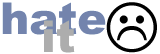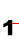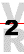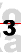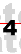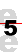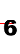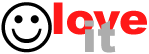```
---------------------------------------------------------------------------
75 Chars Wide```
We're testing ads. Send feedback if you have opinions about this.